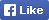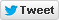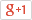Computer/Electronics Questions and Answers
C, C++, VC++, COM/DCOM, DLL and moreLoginSign upHome Electronics Computer Questions Member's Area News Site Map About

Write a program using functions to print armstrong series numbers.

Source Code

 1 #include  2 3 int is_armstrong (int num) 4 { 5 int sum = 0, temp, remainder; 6 temp = num; 7 8 while( temp != 0 ) 9 { 10 /* Get the digit */ 11 remainder = temp % 10; 12 /* calculate cube and sum up */ 13 sum = sum + (remainder * remainder * remainder); 14 /* Shift right by one digit */ 15 temp = temp / 10; 16 } 17 18 if ( num == sum ) { 19 return 1; /* armstrong number */ 20 } else { 21 return 0; /* not armstrong number */ 22 } 23 } 24 25 /* Printing Armstrong number series */ 26 int main(int argc, char *argv[]) 27 { 28 int range; 29 printf ("Enter Max range: "); 30 scanf ("%d", &range); 31 printf ("Printing armstrong numbers from 0 - %d\n", range); 32 for (number = 0; number <= range; number++) { 33 if ( is_armstrong(number) ) { 34 printf ("%d, ", number); 35 } 36 } 37 return 0; 38 } 39

Output

 1 Enter Max range: 15000 2 Printing armstrong numbers from 0 - 15000 3 0, 1, 153, 370, 371, 407,

Find More from our code collection
Armstrong number, binary number to a decimal number, bubble sort, decimal number to binary number, factorial of the given number factors, fibonacci numbers, HCF and LCM, matrix, mergesort, salary of the employee. palindrome, quadratic equation, star patterns, series etc. and much more...Return to Example Source Code